# cv2.findContours() - 轮廓提取算法 -- OpenCV

OpenCV 中轮廓提取函数 findContours() 实现的是这篇论文的算法：

Satoshi Suzuki and others. Topological structural analysis of digitized binary images by border following. Computer Vision, Graphics, and Image Processing, 30(1):32–46, 1985.

## 轮廓

### 目标

• 了解轮廓概念
• 寻找并绘制轮廓
• OpenCV 函数：cv2.findContours(), cv2.drawContours()

### 教程

#### 啥叫轮廓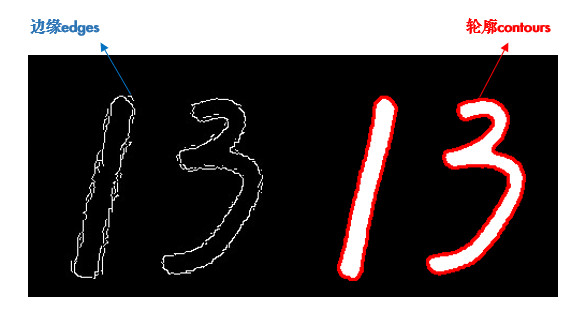#### 寻找轮廓

```import cv2

img_gray = cv2.cvtColor(img, cv2.COLOR_BGR2GRAY)
ret, thresh = cv2.threshold(img_gray, 0, 255, cv2.THRESH_BINARY_INV + cv2.THRESH_OTSU)

# 寻找二值化图中的轮廓
contours, hierarchy = cv2.findContours(
thresh, cv2.RETR_TREE, cv2.CHAIN_APPROX_SIMPLE)
print(len(contours))  # 结果应该为 2```

• 参数2：轮廓的查找方式，一般使用cv2.RETR_TREE，表示提取所有的轮廓并建立轮廓间的层级。更多请参考：RetrievalModes
• 参数3：轮廓的近似方法。比如对于一条直线，我们可以存储该直线的所有像素点，也可以只存储起点和终点。使用cv2.CHAIN_APPROX_SIMPLE就表示用尽可能少的像素点表示轮廓。更多请参考：ContourApproximationModes
• 简便起见，这两个参数也可以直接用真值3和2表示。#### 绘制轮廓

`cv2.drawContours(img, contours, -1, (0, 0, 255), 2)`

```cnt = contours
cv2.drawContours(img, [cnt], 0, (0, 0, 255), 2)```

## 轮廓特征

### 目标

• 计算物体的周长、面积、质心、最小外接矩形等
• OpenCV 函数：cv2.contourArea(), cv2.arcLength(), cv2.approxPolyDP() 等

### 教程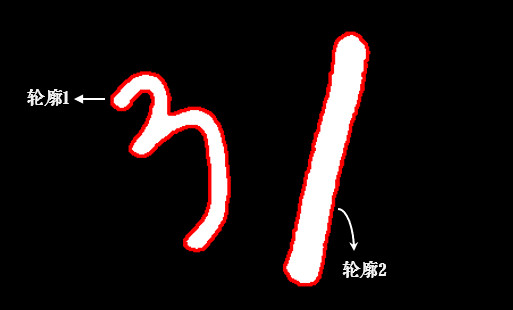```import cv2
import numpy as np

_, thresh = cv2.threshold(img, 0, 255, cv2.THRESH_BINARY_INV + cv2.THRESH_OTSU)
contours, hierarchy = cv2.findContours(thresh, 3, 2)

# 以数字 3 的轮廓为例
cnt = contours```

```img_color1 = cv2.cvtColor(image, cv2.COLOR_GRAY2BGR)
img_color2 = np.copy(img_color1)
cv2.drawContours(img_color1, [cnt], 0, (0, 0, 255), 2)```

#### 轮廓面积

`area = cv2.contourArea(cnt)  # 4386.5`

#### 轮廓周长

`perimeter = cv2.arcLength(cnt, True)  # 585.7`

#### 图像矩

`M = cv2.moments(cnt)`

M 中包含了很多轮廓的特征信息，比如 M['m00'] 表示轮廓面积，与前面 cv2.contourArea() 计算结果是一样的。质心也可以用它来算：

``cx, cy = M['m10'] / M['m00'], M['m01'] / M['m00']  # (205, 281)``

#### 外接矩形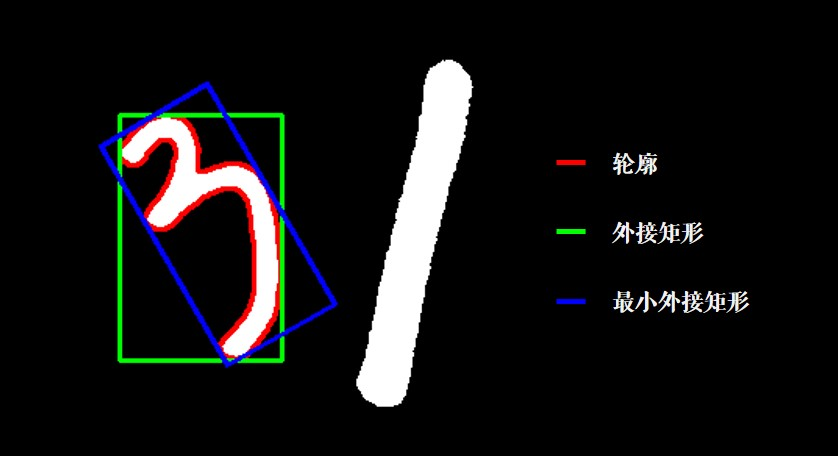```x, y, w, h = cv2.boundingRect(cnt)  # 外接矩形
cv2.rectangle(img_color1, (x, y), (x + w, y + h), (0, 255, 0), 2)

rect = cv2.minAreaRect(cnt)  # 最小外接矩形
box = np.int0(cv2.boxPoints(rect))  # 矩形的四个角点取整
cv2.drawContours(img_color1, [box], 0, (255, 0, 0), 2)```

#### 最小外接圆

```(x, y), radius = cv2.minEnclosingCircle(cnt)
cv2.circle(img_color2, (x, y), radius, (0, 0, 255), 2)```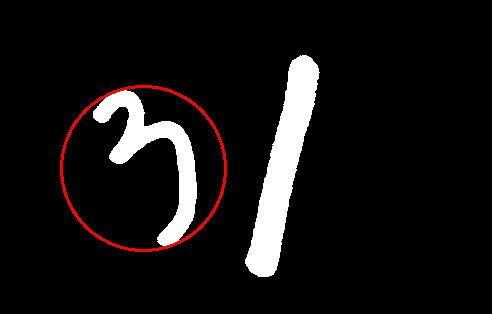#### 拟合椭圆

```ellipse = cv2.fitEllipse(cnt)
cv2.ellipse(img_color2, ellipse, (255, 255, 0), 2)```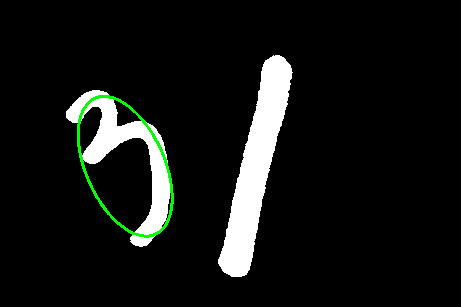#### 形状匹配

cv2.matchShapes() 可以检测两个形状之间的相似度，返回值越小，越相似。先读入下面这张图片：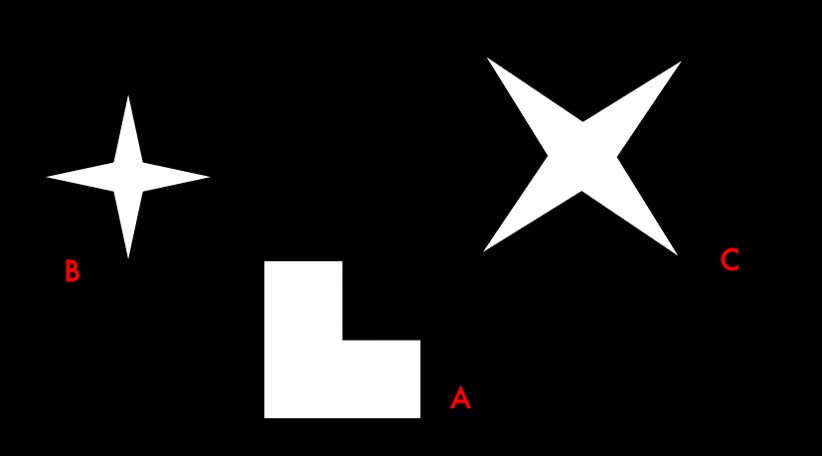```img = cv2.imread('shapes.jpg', 0)
_, thresh = cv2.threshold(img, 0, 255, cv2.THRESH_BINARY + cv2.THRESH_OTSU)
contours, hierarchy = cv2.findContours(thresh, 3, 2)
img_color = cv2.cvtColor(thresh, cv2.COLOR_GRAY2BGR)  # 用于绘制的彩色图```

```cnt_a, cnt_b, cnt_c = contours, contours, contours
print(cv2.matchShapes(cnt_b, cnt_b, 1, 0.0))  # 0.0
print(cv2.matchShapes(cnt_b, cnt_c, 1, 0.0))  # 2.17e-05
print(cv2.matchShapes(cnt_b, cnt_a, 1, 0.0))  # 0.418```

• 参数 3 是匹配方法，详情可参考：ShapeMatchModes
• 参数 4 是 OpenCV 的预留参数，暂时没有实现，可以不用理会。

### 练习

• 前面我们是对图片中的数字3进行轮廓特征计算的，大家换成数字1看看。
• （选做）用形状匹配比较两个字母或数字（这相当于很简单的一个OCR噢）。

### 小结

• cv2.contourArea() 算面积，cv2.arcLength() 算周长，cv2.boundingRect() 算外接矩。
• cv2.minAreaRect() 算最小外接矩，cv2.minEnclosingCircle() 算最小外接圆。
• cv2.matchShapes() 进行形状匹配。

## reference

https://zhuanlan.zhihu.com/p/107257870